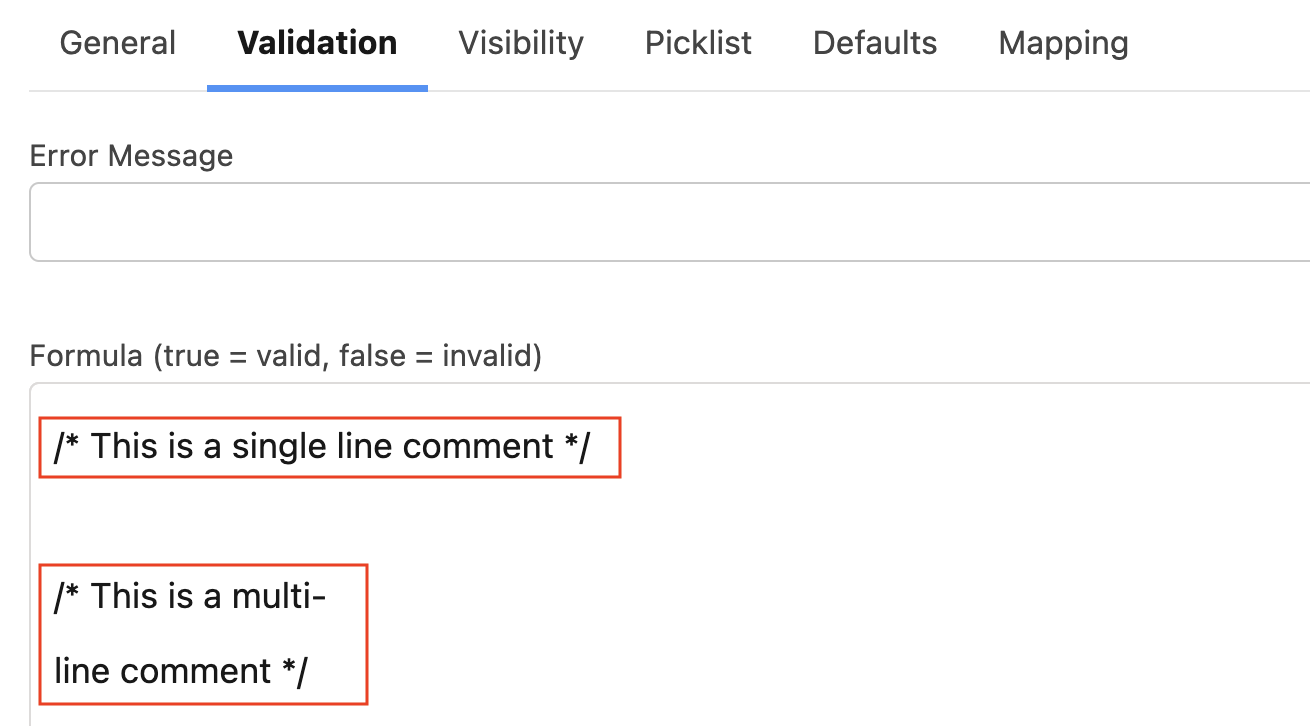List of Supported Operators:

• +
• -
• *
• ^
• /
• !=
• <>
• >
• <
• >=
• <=
• ==
• =
• &&
• ||
• &
• -
• !
• ~, +

List of Supported Formulas:

• DAY
• MONTH
• NOW
• TODAY
• WEEKDAY
• YEAR
• AND
• BLANKVALUE
• CASE
• DATETIMEVALUE
• IF
• ISBLANK
• ISNULL
• ISNUMBER
• NOT
• NULLVALUE
• OR
• ABS
• CEILING
• EXP
• FLOOR
• LN
• LOG
• MAX
• MCEILING
• MFLOOR
• MIN
• MOD
• ROUND
• SQRT
• BEGINS
• CONTAINS
• FIND
• LEFT
• LEN
• LOWER
• MID
• RIGHT
• SUBSTITUTE
• TEXT
• TRIM
• UPPER
• VALUE
• REGEX

For more information on how to create Formulas, please visit this Salesforce webpage that breaks down all the different functionalities: Formula Operators and Functions.